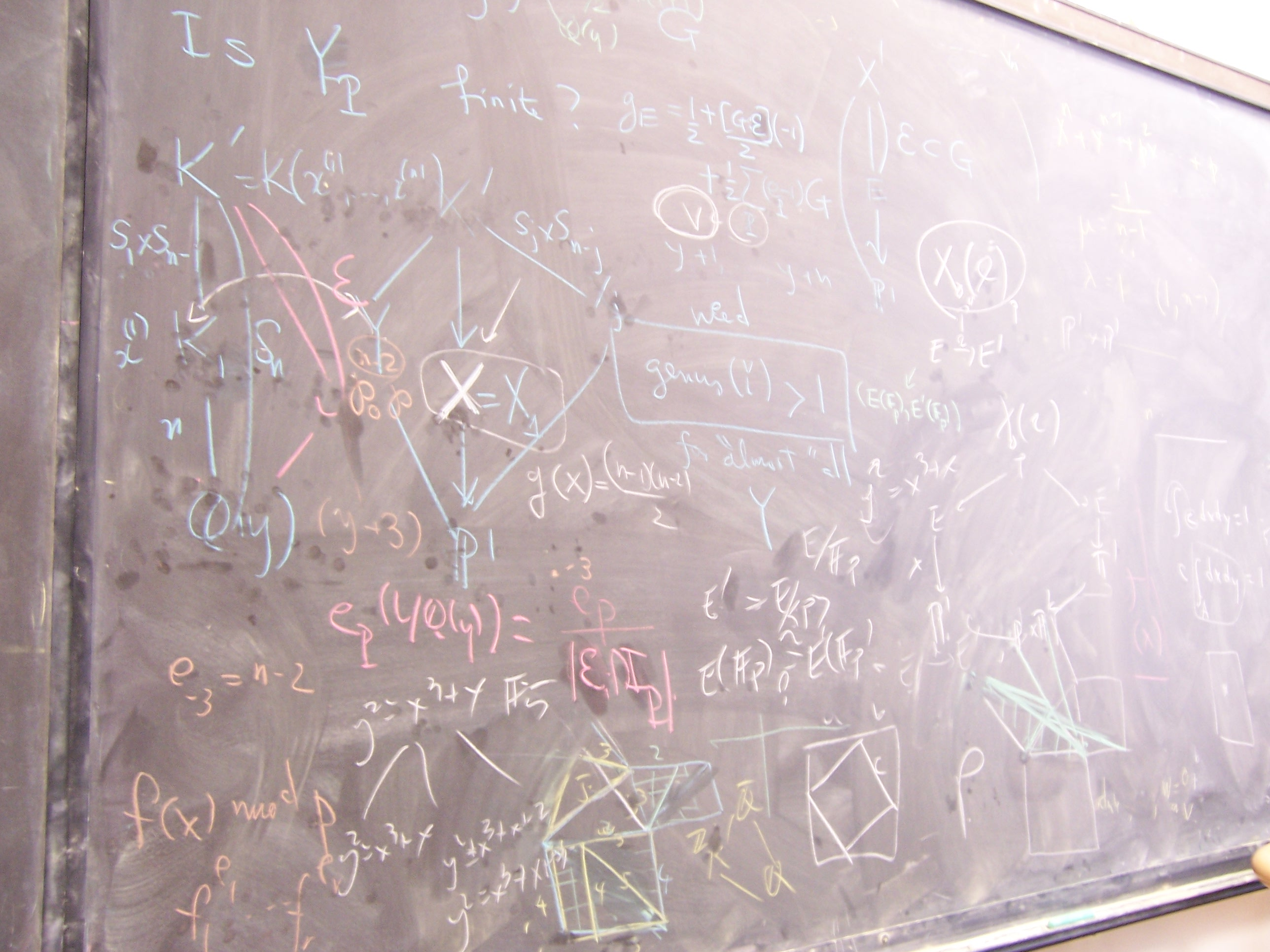John Cullinan

Bard College23. The probability that an elliptic curve has a point of order m, m \in {5, 7, 9} (w/ M. Kenney).
In Preparation.

22. Divisibility of torsion subgroups of abelian surfaces over number fields.
In Preparation.

21. On a probabilistic local-global principal for torsion on elliptic curves (w/ J. Voight).
In Preparation.

20. Fixed-Point Subgroups of GL(3,q).
To appear in Journal of Group Theory.

19. On the Jacobians of curves defined by the Generalized Laguerre Polynomials.
To appear in Experimental Mathematics.

18. Voting with partially ordered preferences (w/ S. Hsiao).
To appear in Mathematics and Social Justice: Perspectives and Resources for the College Classroom, MAA Edited Volume.

17. A remark on the group structure of elliptic curves in towers of finite fields.
New York Journal of Mathematics. 24 857-865 (2018)

16. Algebraic properties of Kaneko-Zagier lifts of supersingular polynomials (w/ F. Hajir).
Proceedings of the American Mathematical Society 145 (2017), no. 6, 2291-2304.

15. Real preimages of duplication on elliptic curves.
Missouri Journal of Mathematical Sciences 29 (2017), no. 1, 19-26.

14. On the Newton polygons of Kaneko-Zagier lifts of supersingular polynomials (w/ R. Gajek-Leonard).
Research in Number Theory 2 (2016), Art. 34

13. On the solution to a generalized Fermat equation: arithmetic and combinatorics (w/ A. Barghi).
Journal of Combinatorics and Number Theory 7 (2015), no. 3, 157-169.

12. Arithmetic properties of generalized Rikuna polynomials (w/ Z. Chonoles et al).
Publications Mathematiques de Besancon: Algebre et Theorie des Nombres, 2014/1, 19-33.

11. On the ranks of Jacobians of curves defined by Jacobi polynomials (w/ A. Etropolski and E. Sell).
Rocky Mountain Journal of Mathematics 44 (2014), no. 1, 23-33.

10. On the Galois groups of Legendre polynomials (w/ F. Hajir).
Indagationes Mathematicae (N.S.) 25 (2014), no. 3, 534-552.

9. A Borda Count procedure for partially ordered ballots (w/ S. Hsiao and D. Polett).
Social Choice Welfare 42 (2014), no. 4, 913-926.

8. Specializations of generalized Rikuna polynomials (w/ C. Cass, A. Rasmussen, and D. Trifunovski).
International Journal of Number Theory 10 (2014), no. 3, 585-600.

7. Primes of prescribed congruence class in short intervals (w/ F. Hajir).
Integers 12 (2012) #A56.

6. Symplectic stabilizers with applications to abelian varieties.
International Journal of Number Theory 8 (2012), no. 2, 321-334.

5. Ramification in iterated towers for rational functions (w/ F. Hajir).
Manuscripta Mathematica 137 (2012), no. 3-4, 273-286.

4. Points of small order on three-dimensional abelian varieties; With an appendix by Yuri Zarhin.
Journal of Algebra 324 (2010), no. 3, 565-577.

3. Algebraic properties of a family of Jacobi polynomials (w/ F. Hajir and E. Sell).
Journal de Theorie des Nombres de Bordeaux 21 (2009), no. 1, 97-108.

2. A computational approach to the 2-torsion structure of an abelian threefold.
Mathematics of Computation 78 (2009), no. 267, 1825-1836.

1. Local-global properties of torsion points on three-dimensional abelian varieties.
Journal of Algebra 311 (2007), no. 2, 736-774